# Excel Multiple Regression (Polynomial Regression)

Share on

Probability and Statistics Index > Excel for Statistics > Excel Multiple Regression

Excel multiple regression can be performed by adding a trendline, or by using the Excel Data Analysis Toolpak. If you just want to know the equation for the line of best fit, adding a trendline will work just fine. If you want to know more detailed information, like the standard error of a sample, then use the Excel Data Analysis Toolpak method.

## Excel Multiple Regression: Adding a Trendline

Example question: Find the equation for the third degree polynomial that fits the following data: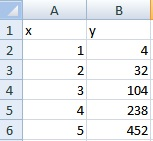Step 1: Type your data into two columns. The x-values should be in one column (i.e. column A); the y-values should be in an adjacent column.

Step 2: Highlight both columns of data.

Step 3: Click “Insert” and then click “Scatter.” Choose the first scatter plot (Scatter with only Markers).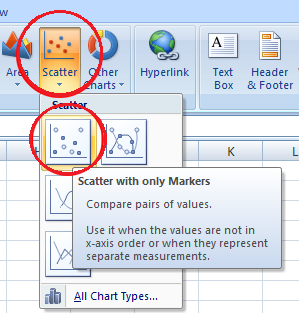Step 4: Click on one of the data points on the graph. This will highlight all the points.

Step 5: Right click, then click “Add trendline.”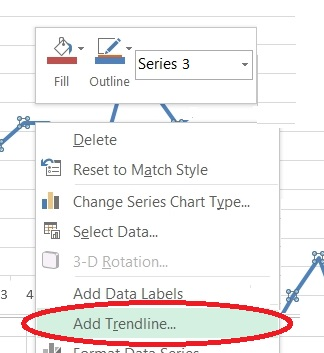Step 6: Click the “Polynomial” radio button. Change the Order to 3.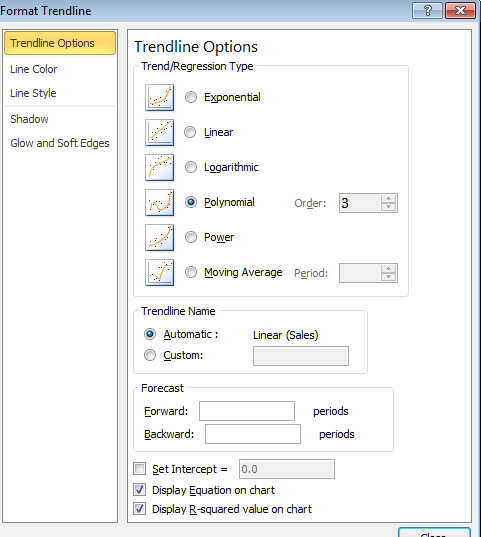Step 7: Click “Display Equation on chart” at the bottom of the pop up window, and then press “Enter.”

Your equation will now show on the chart: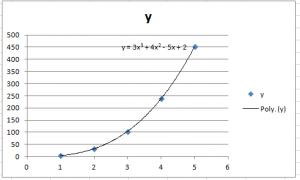Tip: If you want to add additional significant figures to your equation’s coefficients, select the equation on the graph and then use the Number function to increase the number of decimal places.

## Excel Multiple Regression: The Data Analysis Toolpak

Step 1: Type your data in (as in step 1 above).

Step 2: Add a second column containing cubes of your x-values. For this example, cube each of the x-values in column “B”.

Step 2: Click the “Data” tab and then click “Data Analysis.”

Step 3: Select BOTH columns (the x-values and their squares) when choosing x-values on the pop up window. Choose the appropriate column for the y-values.

Step 4: Check the labels box if you have column headers.

Step 5: Select the Confidence Level and Residuals boxes.

Step 6: Select an output area. Press OK.

For help with understanding what the output means, see: Excel Regression Analysis Output Explained.

CITE THIS AS:
Stephanie Glen. "Excel Multiple Regression (Polynomial Regression)" From StatisticsHowTo.com: Elementary Statistics for the rest of us! https://www.statisticshowto.com/excel-multiple-regression/
---------------------------------------------------------------------------Need help with a homework or test question? With Chegg Study, you can get step-by-step solutions to your questions from an expert in the field. Your first 30 minutes with a Chegg tutor is free!